# 一步一步实现Vue的响应式(对象观测)_良笙_前端开发者

watch是平时开发中使用率非常高的功能，其目的是观测一个数据，当数据变化时执行我们预先定义的回调。使用方式如下：

```{
watch: {
obj(val, oldVal) {
console.log(val, oldVal);
}
}
}```

```function watch (data, key, cb) {
// do something
}```
1. watch函数接收3个属性，分别是
2. data: 被观测对象 key: 被观测的属性
3. cb: 数据变化后要执行的回调

Object.defineProperty

```function defineReactive(data, key) {
let val = data[key];

Object.defineProperty(data, key, {
configurable: true,
enumerable: true,
get: function() {
return val;
},
set: function(newVal) {
if (newVal === val) {
return;
}

val = newVal;
}
});
}```

defineReactive函数为data对象的key属性定义了get、set，get返回属性key的值val，set中修改key的值为新值newVal。到目前为止，key属性还是没有什么特殊之处。

`let target = null;`

```function watch(data, key, cb) {
target = cb;
}```

watch函数中target被修改了，但我要是再想调用watch函数一次，也就是说我想在data[key]被修改时，执行两个不同的回调，又或者说，我想再观测data的其它属性，那该怎么办？必须得在target被再次修改前，将其值保存到别处。因为，target是同个属性的不同回调或不同属性的回调所共有的。

```function defineReactive(data, key) {
let val = data[key];
const dep = [];

Object.defineProperty(data, key, {
configurable: true,
enumerable: true,
get: function() {
target && dep.push(target);

return val;
},
set: function(newVal) {
if (newVal === val) {
return;
}

dep.forEach(fn => fn(newVal, val));

val = newVal;
}
});
}```

```function watch(data, key, cb) {
target = cb;
data[key];
target = null;
}```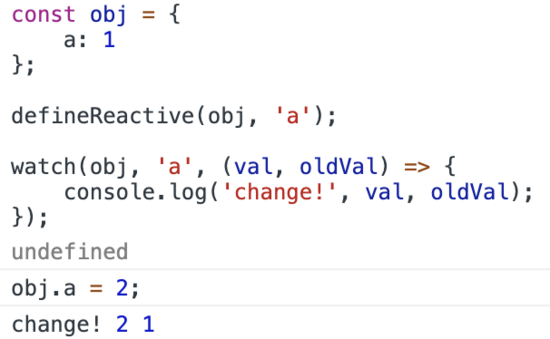Watcher

```class Watcher {
constructor(data, key, cb) {
this.vm = data;
this.key = key;
this.cb = cb;
this.value = this.get();
}

get() {
Dep.target = this;
const value = this.vm[this.key];
Dep.target = null;

return value;
}

update() {
const oldValue = this.value;
this.value = this.vm[this.key];

this.cb.call(this.vm, this.value, oldVal);
}
}```

Dep

Dep的职责就是构建属性key与依赖Watcher之间的联系，其实例一定有一个独一无二的属于属性key的依赖收集框：

```class Dep {
constructor() {
this.subs = [];
}

this.subs.push(sub);
}

depend() {
}

notify() {
for (let sub of subs) {
sub.update();
}
}
}```

subs就是依赖收集框，当属性值被读取时，在depend方法中将依赖收入到框内；当属性值被修改时，在notify方法中将依赖收集框遍历，每一个依赖的update方法都将被执行。

Observer

defineReactive函数只做了一件事，将数据转换成响应式的，我们定义一个Observer类来聚合其功能：

```class Observer {
constructor(data, key) {
this.value = data;

defineReactive(data, key);
}
}

function defineReactive(data, key) {
let val = data[key];
const dep = new Dep();

Object.defineProperty(data, key, {
configurable: true,
enumerable: true,
get: function() {
dep.depend();

return val;
},
set: function(newVal) {
if (newVal === val) {
return;
}

dep.notify();

val = newVal;
}
});
}```

dep不再是一个纯粹的数组，而是一个Dep类的实例。get函数中的依赖收集、set函数中的依赖触发的逻辑，分别用dep.depend、dep.update替代，这让defineReactive函数逻辑变得变得更加清晰。但是Observer类只是在构造函数中调用defineReactive函数，没起什么作用？这当然都是为后面做铺垫的！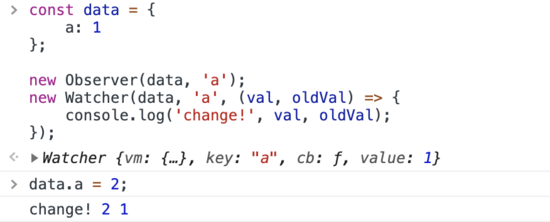```class Observer {
constructor(data) {
this.value = data;

if (isPlainObject(data)) {
this.walk(data);
}
}

walk(value) {
const keys = Object.keys(value);

for (let key of keys) {
defineReactive(value, key);
}
}
}

function isPlainObject(obj) {
return ({}).toString.call(obj) === '[object Object]';
}```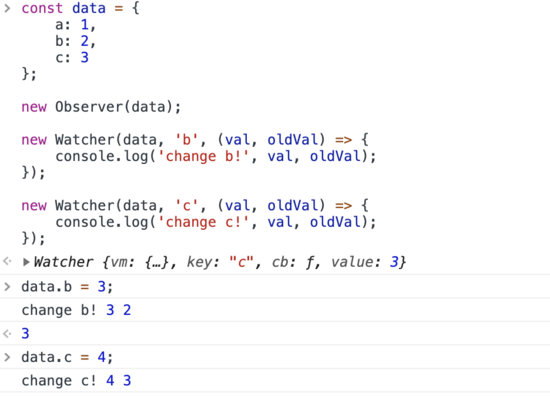```class Observer {
constructor(data) {
this.value = data;

if (isPlainObject(data)) {
this.walk(data);
}
}

walk(value) {
const keys = Object.keys(value);

for (let key of keys) {
const val = value[key];

if (isPlainObject(val)) {
this.walk(val);
}
else {
defineReactive(value, key);
}
}
}
}```

```class Watcher {
constructor(data, path, cb) {
this.vm = data;
this.cb = cb;
this.getter = parsePath(path);
this.value = this.get();
}

get() {
Dep.target = this;
const value = this.getter.call(this.vm);
Dep.target = null;

return value;
}

update() {
const oldValue = this.value;
this.value = this.getter.call(this.vm, this.vm);

this.cb.call(this.vm, this.value, oldValue);
}
}

function parsePath(path) {
if (/.\$_/.test(path)) {
return;
}

const segments = path.split('.');

return function(obj) {
for (let segment of segments) {
obj = obj[segment]
}

return obj;
}
}```

Watcher类实例新增了getter属性，其值为parsePath函数的返回值，在parsePath函数中，返回的是一个匿名函数，匿名函数接收一个参数obj，最后又将obj作为返回值返回，那么这里的重点是匿名函数对obj做了什么处理。

```obj = {
a: {
b: {
c: 1
}
}
}```

`obj = 1````get() {
Dep.target = this;
const value = this.getter.call(this.vm);
Dep.target = null;

return value;
}```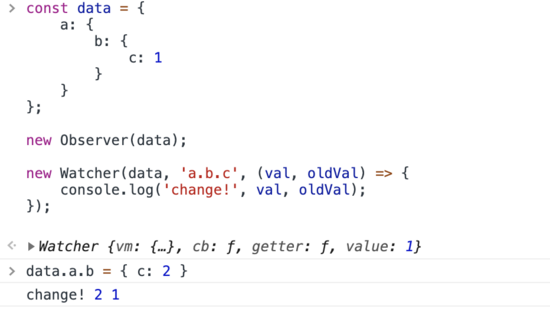vue中，\$watch方法的第一个参数是可以传函数的：

```this.\$watch(() => {
return this.a + this.b;
}, (val, oldVal) => {
console.log(val, oldVal);
});```

```class Watcher {
constructor(data, pathOrFn, cb) {
this.vm = data;
this.cb = cb;
this.getter = typeof pathOrFn === 'function' ? pathOrFn : parsePath(pathOrFn);
this.value = this.get();
}

...

update() {
const oldValue = this.value;
this.value = this.get();

this.cb.call(this.vm, this.value, oldValue);
}
}```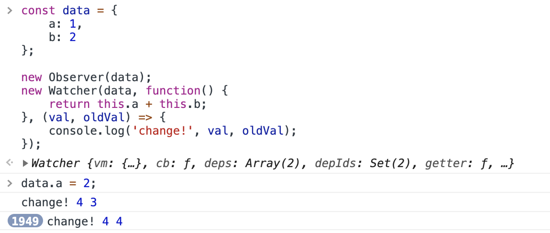```let uid = 1;

class Watcher {
constructor(data, pathOrFn) {
this.id = uid++;
...
}
}

class Dep() {
construct() {
this.subs = [];
this.subIds = new Set();
}
...
const id = sub.id;

if (!this.subIds.has(id)) {
this.subs.push(sub);
}
}
...
}```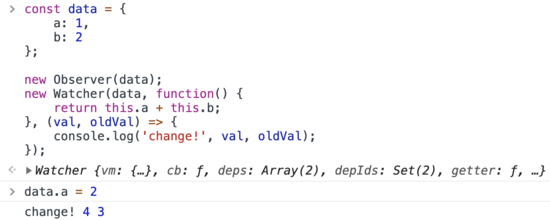```<template>
<div>
<p>{{ a }}</p>
<p>{{ a }}</p>
<p>{{ a }}</p>
</div>
</template>```

64K